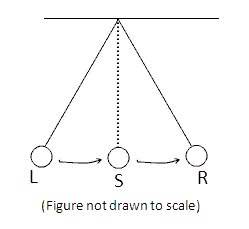# Period of a Pendulum

MoniMini
I am getting confused regarding what a 'period' in a pendulum is.
In a recent Olympiad/Exam I was given this question.....
Can you please give me the method in which it can be done
so that I can solve these type of questions with ease if they
appear in future.....
ThanksA Pendulum oscillates between positions L and R as shown in the figure. It takes 1.2 s to go from L to R and back to the mean position S what is the PERIOD of the pendulum?From my point of view the answer should be 1.6 seconds.....
that's what I wrote as an answer but i'm not very
sure so I just want to confirm....Thanks a Lot!Last edited:

## Answers and Replies

Homework Helper
The period of the pendulum it the time it takes to complete a single swing.

I know that sounds obvious - but when you get confused you should return to the actual physics of the situation and then build the math.

That is a really bad picture :)
A whole swing goes from L to R then back to L again.
(if it starts at L that is)

L -> R is half a period
L -> S is a quarter period.

So if that was your reasoning, you did well.

Extend yourself:
If you put an additional point P half-way between L and S, what proportion of the period is the time to swing from L to P?

MoniMini
Thnx a Lot Simon Sir!
I didn't know what a period is (i'm just in the 6th grade :P) so I
assumed it is something like L - S - R - S - L.......
Thnx for the clarification